Search by Topic

Resources tagged with Factors and multiples similar to Biscuit Decorations:

Filter by: Content type:
Age range:
Challenge level:

There are 114 results

Broad Topics > Numbers and the Number System > Factors and multiplesBiscuit Decorations

Age 5 to 7 Challenge Level:

Andrew decorated 20 biscuits to take to a party. He lined them up and put icing on every second biscuit and different decorations on other biscuits. How many biscuits weren't decorated?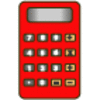Constant Counting

Age 5 to 7 Challenge Level:

You can make a calculator count for you by any number you choose. You can count by ones to reach 24. You can count by twos to reach 24. What else can you count by to reach 24?Tables Teaser

Age 5 to 7 Challenge Level:

How will you work out which numbers have been used to create this multiplication square?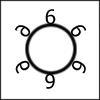Six in a Circle

Age 5 to 7 Challenge Level:

If there is a ring of six chairs and thirty children must either sit on a chair or stand behind one, how many children will be behind each chair?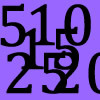Doubling Fives

Age 5 to 7 Challenge Level:

This activity focuses on doubling multiples of five.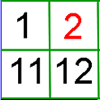What's Left?

Age 5 to 7 Challenge Level:

Use this grid to shade the numbers in the way described. Which numbers do you have left? Do you know what they are called?What's in the Box?

Age 7 to 11 Challenge Level:

This big box multiplies anything that goes inside it by the same number. If you know the numbers that come out, what multiplication might be going on in the box?What Two ...?

Age 7 to 11 Short Challenge Level:

56 406 is the product of two consecutive numbers. What are these two numbers?Ducking and Dividing

Age 7 to 11 Challenge Level:

Your vessel, the Starship Diophantus, has become damaged in deep space. Can you use your knowledge of times tables and some lightning reflexes to survive?The Set of Numbers

Age 5 to 7 Challenge Level:

Can you place the numbers from 1 to 10 in the grid?Grouping Goodies

Age 5 to 7 Challenge Level:

Pat counts her sweets in different groups and both times she has some left over. How many sweets could she have had?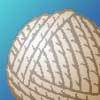Mrs Trimmer's String

Age 5 to 7 Challenge Level:

Can you help the children in Mrs Trimmer's class make different shapes out of a loop of string?Give Me Four Clues

Age 7 to 11 Challenge Level:

Four of these clues are needed to find the chosen number on this grid and four are true but do nothing to help in finding the number. Can you sort out the clues and find the number?Divide it Out

Age 7 to 11 Challenge Level:

What is the lowest number which always leaves a remainder of 1 when divided by each of the numbers from 2 to 10?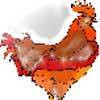Age 5 to 7 Challenge Level:

On a farm there were some hens and sheep. Altogether there were 8 heads and 22 feet. How many hens were there?Skip Counting

Age 5 to 7 Challenge Level:

Find the squares that Froggie skips onto to get to the pumpkin patch. She starts on 3 and finishes on 30, but she lands only on a square that has a number 3 more than the square she skips from.A Mixed-up Clock

Age 7 to 11 Challenge Level:

There is a clock-face where the numbers have become all mixed up. Can you find out where all the numbers have got to from these ten statements?One of Thirty-six

Age 5 to 7 Challenge Level:

Can you find the chosen number from the grid using the clues?What Do You Need?

Age 7 to 11 Challenge Level:

Four of these clues are needed to find the chosen number on this grid and four are true but do nothing to help in finding the number. Can you sort out the clues and find the number?Number Tracks

Age 7 to 11 Challenge Level:

Ben’s class were cutting up number tracks. First they cut them into twos and added up the numbers on each piece. What patterns could they see?Odds and Threes

Age 7 to 11 Challenge Level:

A game for 2 people using a pack of cards Turn over 2 cards and try to make an odd number or a multiple of 3.Spelling Circle

Age 7 to 11 Challenge Level:

Find the words hidden inside each of the circles by counting around a certain number of spaces to find each letter in turn.Multiplication Series: Number Arrays

Age 5 to 11

This article for teachers describes how number arrays can be a useful reprentation for many number concepts.Crossings

Age 7 to 11 Challenge Level:

In this problem we are looking at sets of parallel sticks that cross each other. What is the least number of crossings you can make? And the greatest?Being Resilient - Primary Number

Age 5 to 11 Challenge Level:

Number problems at primary level that may require resilience.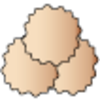Lots of Biscuits!

Age 5 to 7 Challenge Level:

Help share out the biscuits the children have made.Surprising Split

Age 7 to 11 Challenge Level:

Does this 'trick' for calculating multiples of 11 always work? Why or why not?Becky's Number Plumber

Age 7 to 11 Challenge Level:

Becky created a number plumber which multiplies by 5 and subtracts 4. What do you notice about the numbers that it produces? Can you explain your findings?Numbers as Shapes

Age 5 to 7 Challenge Level:

Use cubes to continue making the numbers from 7 to 20. Are they sticks, rectangles or squares?Money Measure

Age 7 to 11 Challenge Level:

How can you use just one weighing to find out which box contains the lighter ten coins out of the ten boxes?Which Is Quicker?

Age 7 to 11 Challenge Level:

Which is quicker, counting up to 30 in ones or counting up to 300 in tens? Why?Tom's Number

Age 7 to 11 Challenge Level:

Work out Tom's number from the answers he gives his friend. He will only answer 'yes' or 'no'.What Is Ziffle?

Age 7 to 11 Challenge Level:

Can you work out what a ziffle is on the planet Zargon?Flashing Lights

Age 7 to 11 Challenge Level:

Norrie sees two lights flash at the same time, then one of them flashes every 4th second, and the other flashes every 5th second. How many times do they flash together during a whole minute?Zios and Zepts

Age 7 to 11 Challenge Level:

On the planet Vuv there are two sorts of creatures. The Zios have 3 legs and the Zepts have 7 legs. The great planetary explorer Nico counted 52 legs. How many Zios and how many Zepts were there?Path to the Stars

Age 7 to 11 Challenge Level:

Is it possible to draw a 5-pointed star without taking your pencil off the paper? Is it possible to draw a 6-pointed star in the same way without taking your pen off?Little Squares

Age 5 to 7 Challenge Level:

Look at the squares in this problem. What does the next square look like? I draw a square with 81 little squares inside it. How long and how wide is my square?In the Money

Age 7 to 11 Challenge Level:

One quarter of these coins are heads but when I turn over two coins, one third are heads. How many coins are there?Multiplication Squares

Age 7 to 11 Challenge Level:

Can you work out the arrangement of the digits in the square so that the given products are correct? The numbers 1 - 9 may be used once and once only.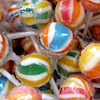Lots of Lollies

Age 5 to 7 Challenge Level:

Frances and Rishi were given a bag of lollies. They shared them out evenly and had one left over. How many lollies could there have been in the bag?Being Collaborative - Primary Number

Age 5 to 11 Challenge Level:

Number problems at primary level to work on with others.It Figures

Age 7 to 11 Challenge Level:

Suppose we allow ourselves to use three numbers less than 10 and multiply them together. How many different products can you find? How do you know you've got them all?Which Numbers? (1)

Age 7 to 11 Challenge Level:

I am thinking of three sets of numbers less than 101. They are the red set, the green set and the blue set. Can you find all the numbers in the sets from these clues?Scoring with Dice

Age 7 to 11 Challenge Level:

I throw three dice and get 5, 3 and 2. Add the scores on the three dice. What do you get? Now multiply the scores. What do you notice?Which Numbers? (2)

Age 7 to 11 Challenge Level:

I am thinking of three sets of numbers less than 101. Can you find all the numbers in each set from these clues?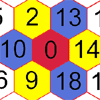Nineteen Hexagons

Age 5 to 7 Challenge Level:

In this maze of hexagons, you start in the centre at 0. The next hexagon must be a multiple of 2 and the next a multiple of 5. What are the possible paths you could take?Factor-multiple Chains

Age 7 to 11 Challenge Level:

Can you see how these factor-multiple chains work? Find the chain which contains the smallest possible numbers. How about the largest possible numbers?Domino Pick

Age 5 to 7 Challenge Level:

Are these domino games fair? Can you explain why or why not?Multiply Multiples 1

Age 7 to 11 Challenge Level:

Can you complete this calculation by filling in the missing numbers? In how many different ways can you do it?The Moons of Vuvv

Age 7 to 11 Challenge Level:

The planet of Vuvv has seven moons. Can you work out how long it is between each super-eclipse?